Next: Correction of the step Up: Model and learning algorithm Previous: Improved approximation of the

## Feedforward and backward computations

The feedforward computations start with the parameters of the posterior approximation of the unknown variables of the model. For the factors, the parameters of the posterior approximation are the posterior mean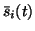, the posterior variance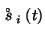and the dependence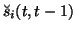. The end result of the feedforward computations is the value of the cost function C.

The first stage of the computations is the iteration of (8) to obtain the marginalised posterior meanand variance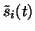of the factors. Thereafter the computations proceed like in the NLFA algorithm: the means and variances are propagated through the MLP networks. The final stage, the computation of the cost function, differs only in the terms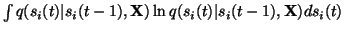and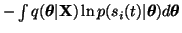. In the NLFA algorithm, the former had the form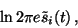(9)

but now they have the form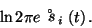(10)

The latter terms can be shown to yield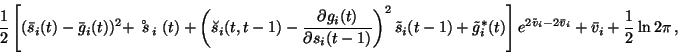(11)

where the ith component of the vector g(s(t-1)) is denoted by gi(t) and the variance parameter of the ith factor by vi, and by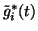, the posterior variance of gi(t)without the contribution of si(t-1), that is, assuming si(t-1)fixed. Notice that ifis zero, the term inside the square brackets takes the form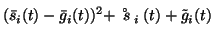becauseis defined to be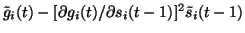.

In the feedbackward phase, the gradient of the cost function Cw.r.t. the parameters of the posterior approximation is computed by the back-propagation algorithm, that is, the steps of the feedforward computations are reversed and the gradient of the cost function is propagated backwards to the parameters of the posterior approximation. Since the essential modification to the feedforward phase of NLFA algorithm is (8), this is also the essential modification in the backward computations.

The cost function is a function of parameters of the posterior approximation. In the computation of the cost function, the marginalised posterior variancesof the factors are used as intermediate variables and hence the gradient is also computed through these variables. Let us use the notation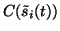to mean that C is considered to be a function of the intermediate variables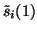,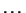,in addition to the parameters of the posterior approximation. The gradient computations resulting from (8) by the chain rule are then as follows: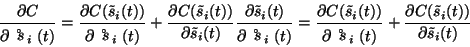(12)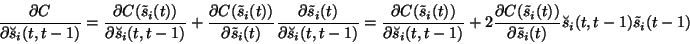(13)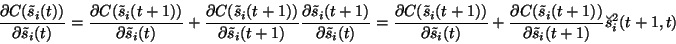(14)

The terms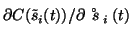and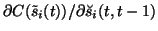can be computed from (10) and (11) while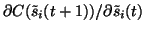also includes terms originating from the mappings f and g as their feedforward computation starts with the posterior meansand variances.

In the adaptation, the posterior meansof the factors are treated as in the NLFA algorithm except for the correction in the step size which is discussed in section 3.3. The variancesare adapted likein the NLFA. The posterior dependenceis adapted by solving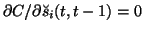which yields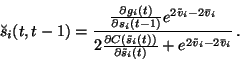(15)

Equation (15) shows thatdepends on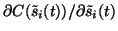which in turn depends on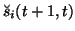as (14) shows. This means that the update of the dependenciesand the computation of the gradient w.r.t. the marginalised varianceare done recursively backward in time which is the counterpart of (8) where the marginalised variances are computed recursively forward in time.Next: Correction of the step Up: Model and learning algorithm Previous: Improved approximation of the
Harri Valpola
2000-10-17